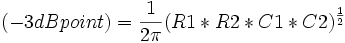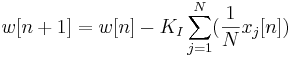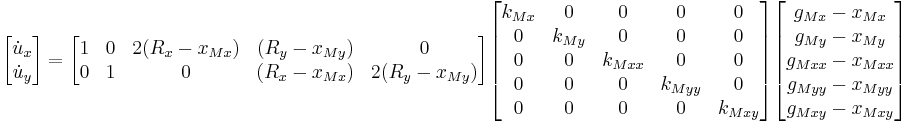# The sandbox

This is the sandbox, so I'm assuming I can edit this as I please.

Test0

# Test1

## test$(-3dB point) = \frac{1}{2\pi}(R1*R2*C1*C2)^\frac{1}{2}$$x[n+1] = x[n] + \gamma(u[n]-x[n]) - K_P\sum_{j=1}^N(\frac{1}{N}x_j[n]) + K_I\sum_{j=1}^N(\frac{1}{N}w_j[n])$$w[n+1] = w[n] - K_I\sum_{j=1}^N(\frac{1}{N}x_j[n])$$\begin{bmatrix} \dot u_x \\ \dot u_y \\ \end{bmatrix} = \begin{bmatrix} 1 & 0 & 2(R_x-x_{Mx}) & (R_y-x_{My}) & 0 \\ 0 & 1 & 0 & (R_x-x_{Mx}) & 2(R_y-x_{My})\\ \end{bmatrix} \begin{bmatrix} k_{Mx} & 0 & 0 & 0 & 0 \\ 0 & k_{My} & 0 & 0 & 0 \\ 0 & 0 & k_{Mxx} & 0 & 0 \\ 0 & 0 & 0 & k_{Myy} & 0 \\ 0 & 0 & 0 & 0 & k_{Mxy} \\ \end{bmatrix} \begin{bmatrix} g_{Mx}-x_{Mx} \\ g_{My}-x_{My}\\ g_{Mxx}-x_{Mxx} \\ g_{Myy}-x_{Myy} \\ g_{Mxy}-x_{Mxy} \\ \end{bmatrix}$

## Testing

Class 1 (W 9/20)

Welcome to the course and course website. Structure of the course (HW due Wed, student-generated lectures and learning materials, in-class assignments, feedback on student lectures, occasional Friday quizzes). Book, software, (lack of) D-H parameters, syllabus, V-REP simulator, office hours.

At home:

Videos: first 3 videos of Chapter 2, through Chapter 2.2
Software: download github software with book, install V-REP and verify that you can use Scenes 1 and 2 (the UR5)
HW1, due 1:30 PM 9/27: Exercises 2.3, 2.9, 2.20, 2.29. Also, create your own example system with closed loops, something not in the book, and solve for the degrees of freedom using Grubler's formula. Make it something that exists or occurs in common experience, not necessarily a robot. Imagine using it to teach someone about Grubler's formula.

Class 2 (F 9/22)

Sample student lecture

At home:

Videos: 2 videos on Chapter 2.3

Class 3 (M 9/25)

Bring your laptop, demo V-REP UR5 scenes
Help with HW1

At home:

Videos: 2 videos, Chapter 2.4 and 2.5
Turn in HW1

Class 4 (W 9/27)

Solutions to HW1; student examples of Grubler's formula

At home:

Videos: first 3 videos of Chapter 3, through Chapter 3.2.1